Warning

This documents an unmaintained version of NetworkX. Please upgrade to a maintained version and see the current NetworkX documentation.

# closeness_centrality¶

closeness_centrality(G, u=None, distance=None, normalized=True)[source]

Compute closeness centrality for nodes.

Closeness centrality  of a node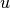is the reciprocal of the sum of the shortest path distances fromto all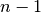other nodes. Since the sum of distances depends on the number of nodes in the graph, closeness is normalized by the sum of minimum possible distances.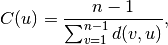where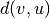is the shortest-path distance between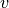and, and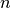is the number of nodes in the graph.

Notice that higher values of closeness indicate higher centrality.

Parameters: G (graph) – A NetworkX graph u (node, optional) – Return only the value for node u distance (edge attribute key, optional (default=None)) – Use the specified edge attribute as the edge distance in shortest path calculations normalized (bool, optional) – If True (default) normalize by the number of nodes in the connected part of the graph. nodes – Dictionary of nodes with closeness centrality as the value. dictionary

Notes

The closeness centrality is normalized to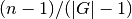whereis the number of nodes in the connected part of graph containing the node. If the graph is not completely connected, this algorithm computes the closeness centrality for each connected part separately.

If the ‘distance’ keyword is set to an edge attribute key then the shortest-path length will be computed using Dijkstra’s algorithm with that edge attribute as the edge weight.

References

  Linton C. Freeman: Centrality in networks: I. Conceptual clarification. Social Networks 1:215-239, 1979. http://leonidzhukov.ru/hse/2013/socialnetworks/papers/freeman79-centrality.pdf